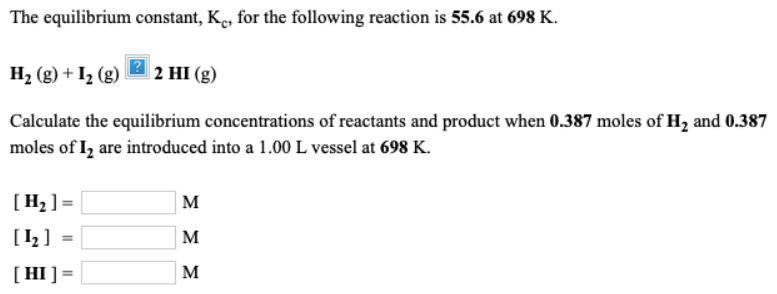# The equilibrium constant, Kc, for the following reaction is 55.6 at 698 K. H2(g) + I2(g) -> 2HI(g) Calculate the equilibrium concentrations of reactants and product when 0.387 moles of H2 and 0.387 moles of I2 are introduced into a 1.00 L vessel at 698 K. [H2] = ___ M [I2] = ___ M [HI] = ___ M